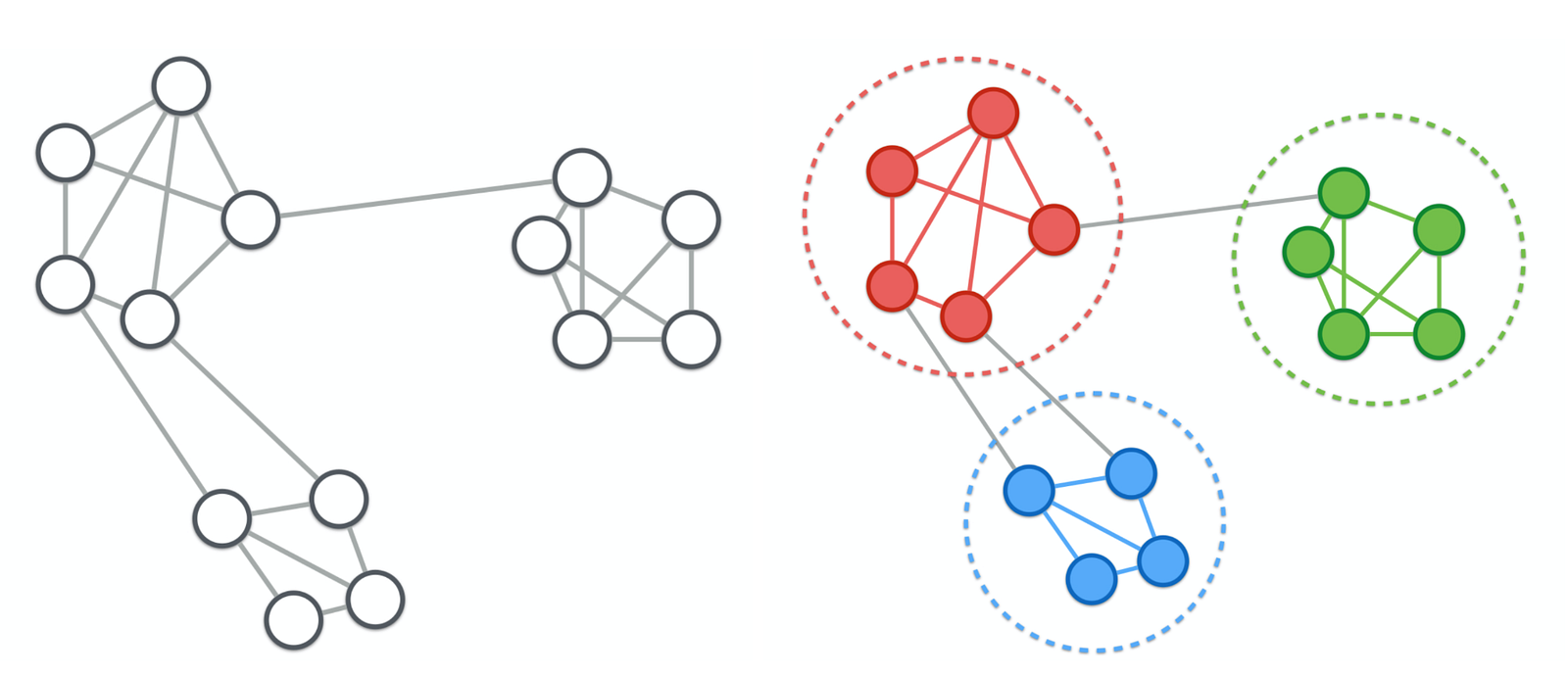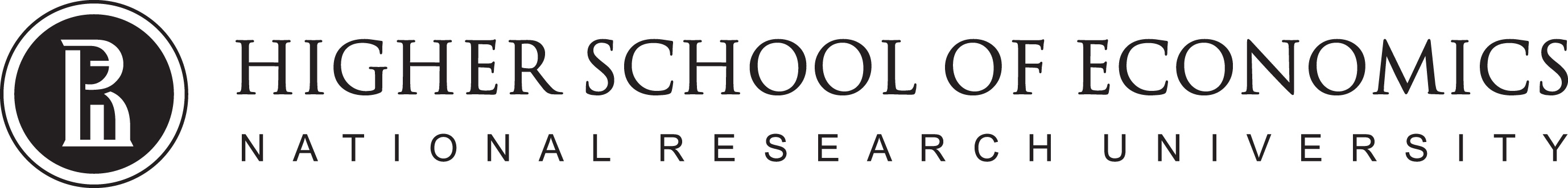# Data Analysis

## Clustering1

1. Some materials are taken from machine learning course of Victor Kitov

# Clustering¶

## Aim of clustering¶

Clustering is partitioning of objects into groups so that:

• inside groups objects are very similar
• objects from different groups are dissimilar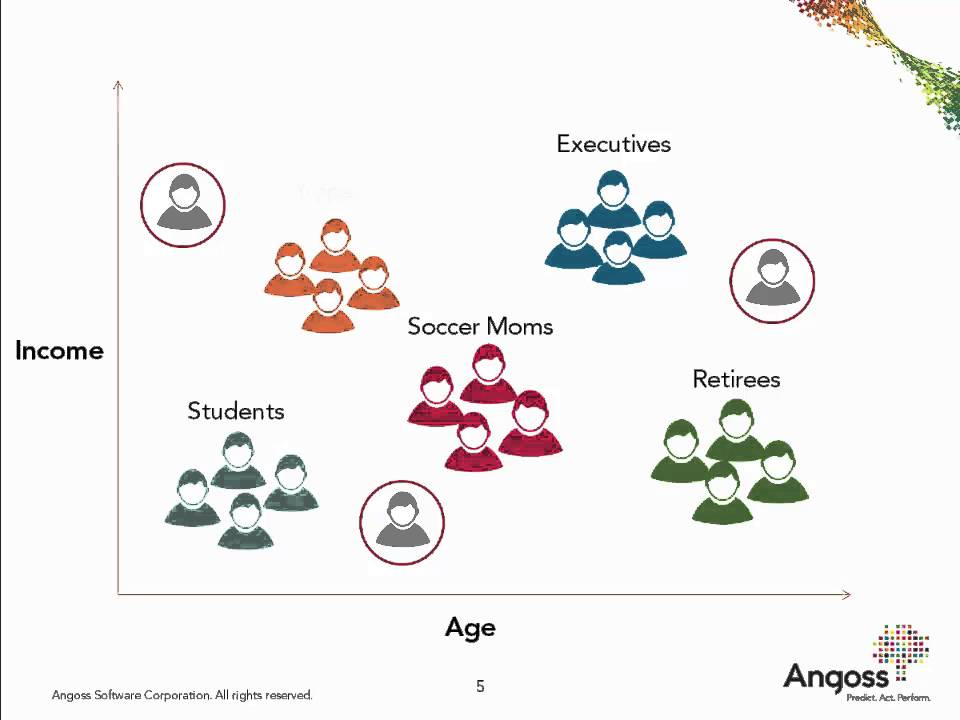## Aim of clustering¶

• Unsupervised learning
• No definition of ''similar''
• different algorithms use different formalizations of similarity

## Applications of clustering¶

• data summarization
• feature vector is replaced by cluster number
• feature extraction
• cluster number, cluster average target, distance to native cluster center / other clusters
• community detection in networks
• nodes - people, similarity - number of connections
• outlier detection
• outliers do not belong any cluster

## Groups of clustering methods¶

• Prototype-based
• Replace cluster with ethalon object
• Hierarchcal
• Build cluster hierarchy
• Density-based
• Find dence groups of objects
• Spectral
• Use spectral techniques
• Grid-based
• Split feature space in grids and connect them
• Probabilistic models
• Assume some mixture of probability distributions

## Clustering algorithms comparison¶

We can compare clustering algorithms in terms of:

• computational complexity
• do they build flat or hierarchical clustering?
• can the shape of clustering be arbitrary?
• if not is it symmetrical, can clusters be of different size?
• can clusters vary in density of contained objects?
• robustness to outliers

# K-means¶

## Prototype-based clustering¶

• Clustering is flat (not hierarchical)
• Number of clusters $K$ is specified in advance
• Each object $x_{n}$ is associated with a cluster $z_{n}$
• $z_{n} \in \{1,\dots,K\}$
• Each cluster $C_{k}$ is defined by its representative $\mu_{k}$, $k=1,2,...K.$
• Criterion to find representatives $\mu_{1},...\mu_{K}$: $$Q(z_{1},...z_{N})=\sum_{n=1}^{N}\min_{k}\rho(x_{n},\mu_{k})\to\min_{\mu_{1},...\mu_{K}}$$

## Generic algorithm¶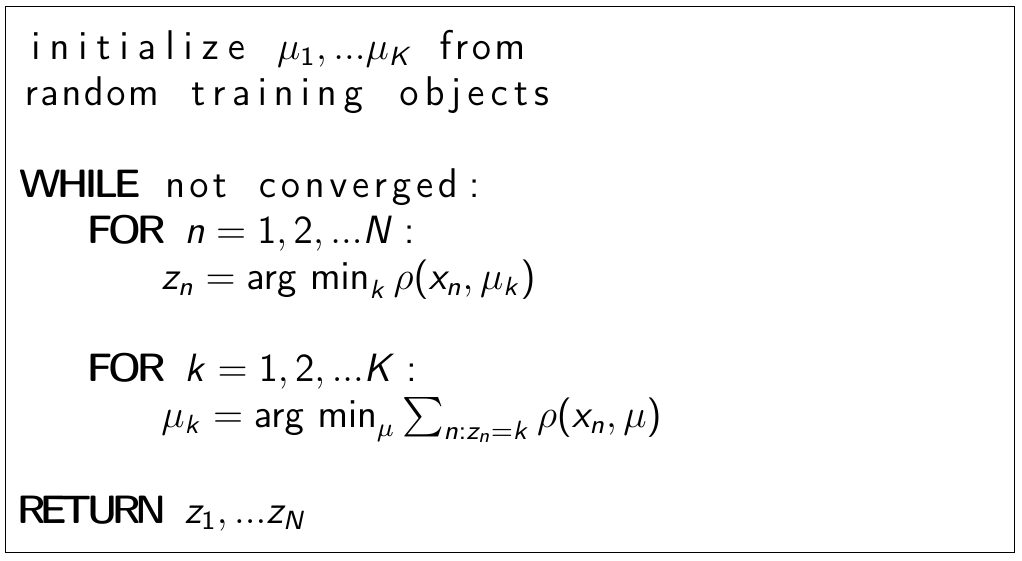• different distance functions lead to different algorithms:

• $\rho(x,x')=\left\lVert x-x'\right\rVert _{2}^{2}$=> K-means
• $\rho(x,x')=\left\lVert x-x'\right\rVert _{1}$=> K-medians
• $\mu_{k}$ may be arbitrary or constrained to be existing objects

• $K$ - is set by user

• if chosen small=>distinct clusters will get merged
• better to take $K$ larger and then merge similar clusters.
• Shape of clusters is defined by $\rho(\cdot,\cdot)$

## Example¶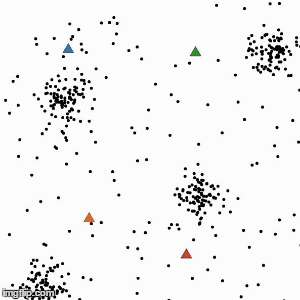## K-means algorithm¶

• Suppose we want to cluster our data into $K$ clusters.
• Cluster $i$ has a center $\mu_{i}$, i=1,2,...K.
• Consider the task of minimizing $$\sum_{n=1}^{N}\left\lVert x_{n}-\mu_{z_{n}}\right\rVert _{2}^{2}\to\min_{z_{1},...z_{N},\mu_{1},...\mu_{K}}\quad (1)$$ where $z_{i}\in\{1,2,...K\}$ is cluster assignment for $x_{i}$ and $\mu_{1},...\mu_{K}$ are cluster centers.
• Direct optimization requires full search and is impractical.
• K-means is a suboptimal algorithm for optimizing (1).

## K-means algorithm¶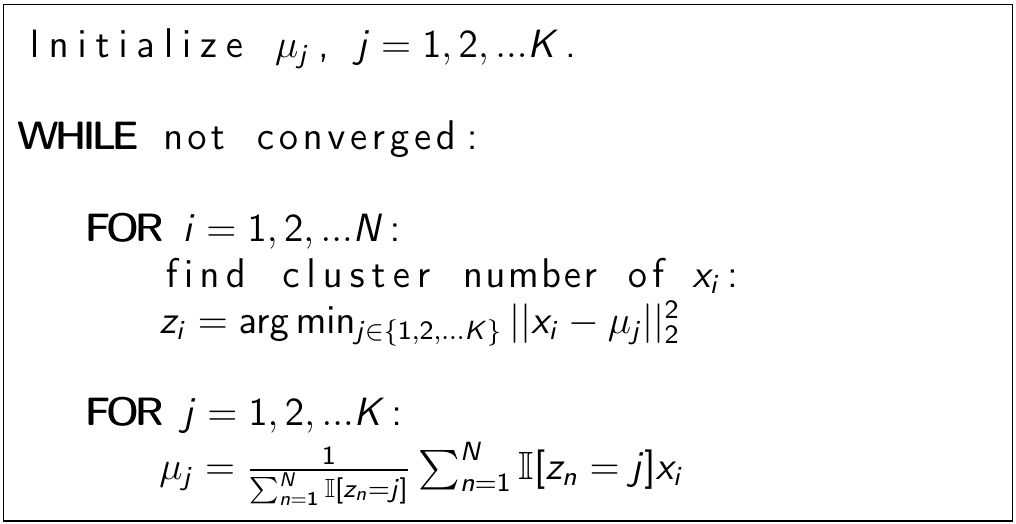## K-means properties¶

• Convergence conditions
• maximum number of iterations reached
• cluster assignments $z_{1},...z_{N}$ stop to change (exact)
• $\{\mu_{i}\}_{i=1}^{K}$ stop changing significantly (approximate)
• Initialization:
• typically $\{\mu_{i}\}_{i=1}^{K}$ are initialized to randomly chosen training objects

## K-means properties¶

• Optimality:
• criteria is non-convex
• solution depends on starting conditions
• may restart several times from different initializations and select solution giving minimal value of (1).
• Complexity: $O(NDKI)$
• $K$ is the number of clusters
• $I$ is the number of iterations.
• usually few iterations are enough for convergence.

## Gotchas¶

• K-means assumes that clusters are convex: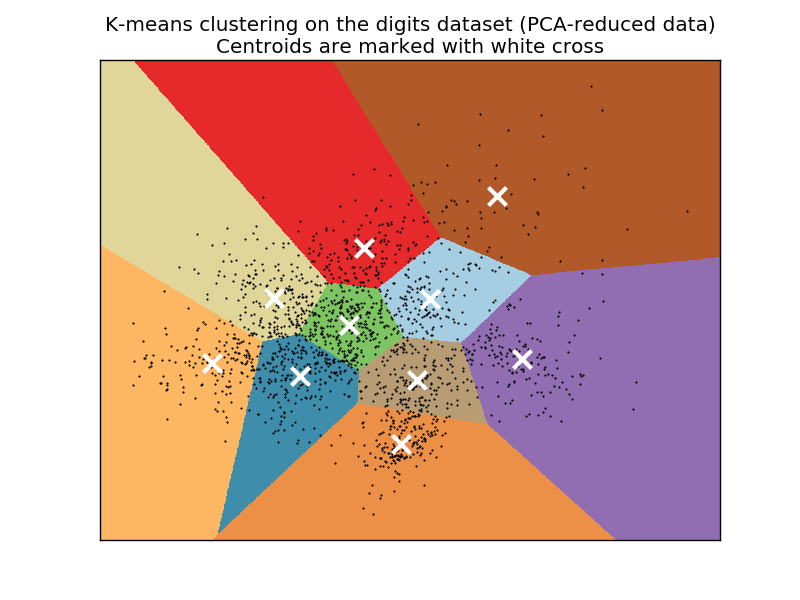• It always finds clusters even if none actually exist
• need to control cluster quality metrics

## Main factors of solution¶

• Initial position of centroids
• Number of centroids

## Number of centroids - K¶

• Don't use vanilla k-means (X-means, ik-means)
• Consider cluster quality measures
• Use heuristics

## Elbow method¶

• Criterion of k-means $$Q(C) = Q(z_{1},...z_{N}) = \sum_{n=1}^{N}\left\lVert x_{n}-\mu_{z_{n}}\right\rVert _{2}^{2}\to\min_{z_{1},...z_{N},\mu_{1},...\mu_{K}}\quad (1)$$
• Lets take all possible values of $K$, and chose one that delivers minimum of $Q(C)$!
• Won't work! Why?

## Elbow method¶

• Choose $K$ after which $Q(C)$ stops decreasing rapidly
• A bit more formally $$D(k) = \frac{|Q^{(k)}(C) - Q^{(k+1)}(C)|}{|Q^{(k-1)}(C) - Q^{(k)}(C)|} \quad \text{" is small "}$$
In :
plot = interact(elbow_demo, k=IntSlider(min=2,max=8,step=1,value=2))


## Warning!¶

• Heuristics are not dogma!
• If at least some clusters are interpretable - this is good

## Centroid initialization¶

• Initiallize randombly
• Run multiple times and take result with smallest $Q(C)$
• Use result of another clustering method as starting point
• k-means++

### K-means++¶

• Choose 1st centroid randomly from initial objects
• For each data point calculate its distance to closest centroid $d_{\min}(x_i) = \min_{\mu_j} \|x_i - \mu_j\|^2$
• Take point as next centroid with probability $p(x_i) \propto d_{\min}(x_i)$
In :
interact(demo_kmpp, iters=IntSlider(min=1,max=6,step=1,value=1))

Out:
<function __main__.demo_kmpp(iters=1)>

## K-means¶

• Not robust to outliers
• K-medians is a bit more robust
• K-representatives may create singleton clusters in outliers if centroids get initialized with outlier
• better to init centroids with mean of $m$ randomly chosen objects
• Constructs spherical clusters of similar radii
• Allows kernel version which can find non-convex clusters in original space

# Hierarchical clustering¶

## Insparation - Motivation¶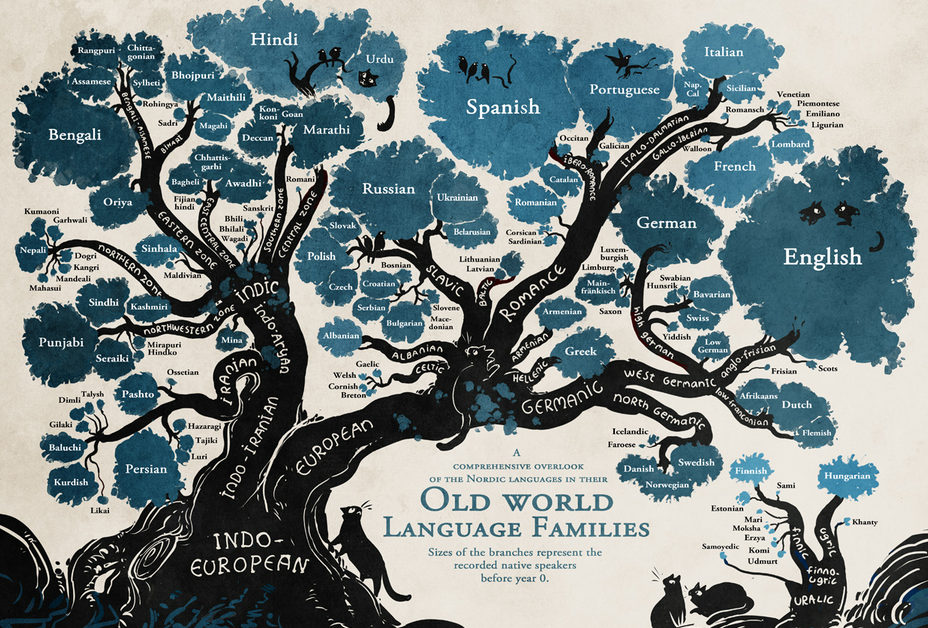## Insparation - Motivation¶

• Number of clusters $K$ not known a priory.
• Clustering is usually not flat, but hierarchical with different levels of granularity:
• sites in the Internet
• books in library
• animals in nature

## Hierarchical clustering¶

Hierarchical clustering may be:

• top-down
• divisive clustering
• bottom-up
• aglomerative clustering

## Aglomerative example¶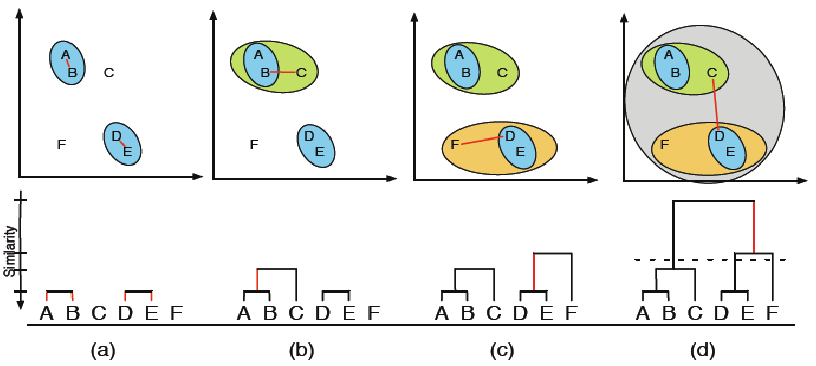## Dendrogram¶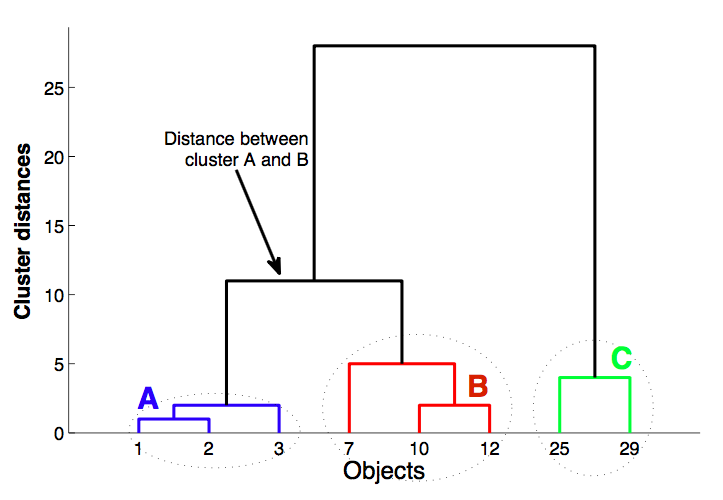# Algorithm¶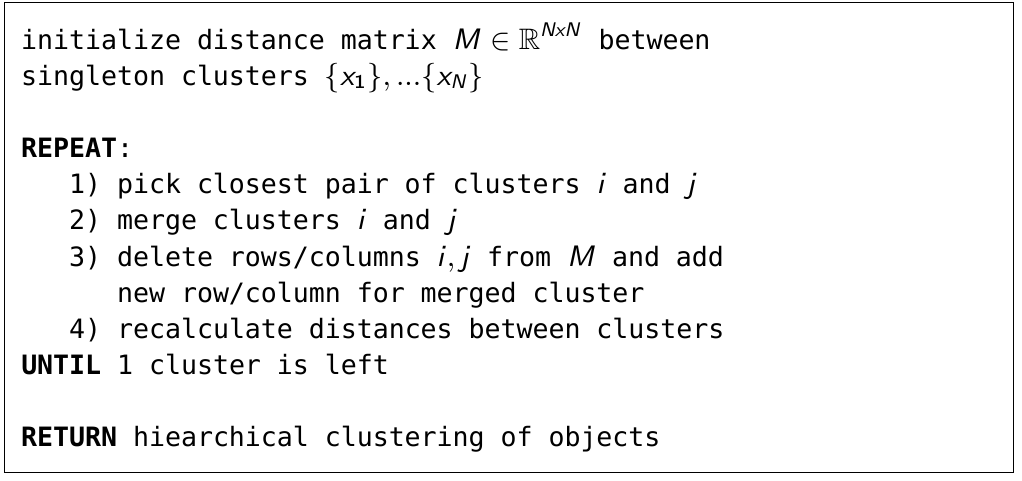• What is "closest pair of clusters"?
• How do we recalculate distances between clusters?

## Distance recalculation¶

• Consider clusters $A=\{x_{i_{1}},x_{i_{2}},...\}$ and $B=\{x_{j_{1}},x_{j_{2}},...\}$.
• We can define the following natural ways to recalculate distances
• nearest neighbour (or single link) $$\rho(A,B)=\min_{a\in A,b\in B}\rho(a,b)$$
• furthest neighbour (or complete link) $$\rho(A,B)=\max_{a\in A,b\in B}\rho(a,b)$$
• group average link $$\rho(A,B)=\frac{1}{N_AN_B}\sum\limits_{a\in A,b\in B}\rho(a,b)$$
• closest centroid (or centroid-link) $$\rho(A,B)=\rho(\mu_{A},\mu_{B})$$ where $\mu_{U}=\frac{1}{|U|}\sum_{x\in U}x$ or $m_{U}=median_{x\in U}\{x\}$

## Lance–Williams formula¶

$$\rho(C_i \cup C_j, C_k) = a_i \cdot \rho(C_i, C_k) + a_j \cdot \rho(C_j, C_k) + b \cdot \rho(C_i, C_j) + c \cdot |\rho(C_i, C_k) - \rho(C_j, C_k)|$$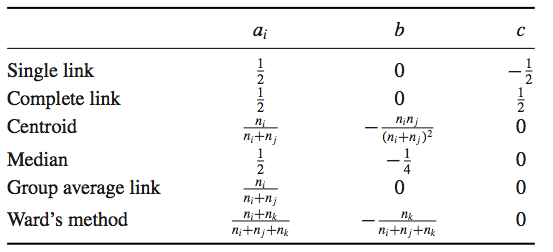## Heuristics for dendrogram quality¶

• cophenetic distance between objects $x_i$ и $x_j$ - height of dendrogram at which whose two objects have merged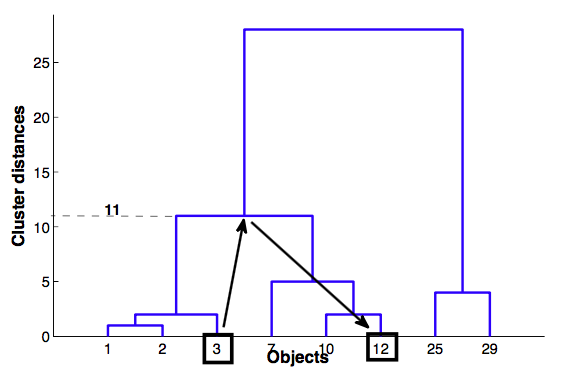### Cophenetic correlation¶

• Cophenetic correlation — correlation between Cophenetic distance and simple distance between objects
$$\text{cophCorr} = \frac{\sum\limits_{i < j}(d(x_i, x_j) - \bar{d})(coph(x_i, x_j) - \bar{coph})}{\sqrt{\sum\limits_{i < j}(d(x_i, x_j) - \bar{d})^2 \cdot \sum\limits_{i < j}(coph(x_i, x_j) - \bar{coph})^2}}$$

If dendrogram is good, correlation should be high

In :
interact(coph_demo, k=IntSlider(min=2, max=10, step=1, value=2), link=['complete', 'single', 'average', 'centroid'], metric=['euclidean', 'cityblock'])

Out:
<function __main__.coph_demo(link='single', metric='euclidean', k=2)>

• Results in full hierarchy of objects
• Various ways to calculate distances
• Nice visualization
• Needs a lot of resources

# DBScan¶

## Goal¶

• Identify clusters as groups of densly connected points, separated by sparse areas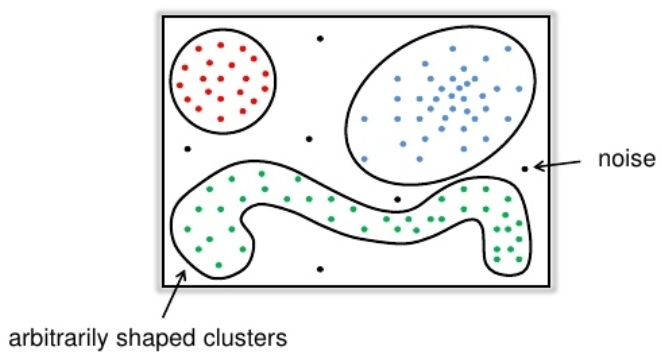## Idea¶

• Identify 2 parameters
• $\epsilon$ - radius of neighbourhood of each point. $N_\epsilon(p)$ - neighbourhood of point $p$
• min_pts - minimal number of points inside neighbourhood
• To start a cluster from point there should be at least min_pts points in $N_\epsilon$
• If this conditions is satisfied - spread cluster and check all points neighbours by the same crierion

## Types of points¶

• Core point: point having $\ge \texttt{min_pts}$ points in its $\varepsilon$ neighbourhood
• Border point: not core point, having at least 1 core point in its $\varepsilon$ neighbourhood
• Noise point: neither a core point nor a border point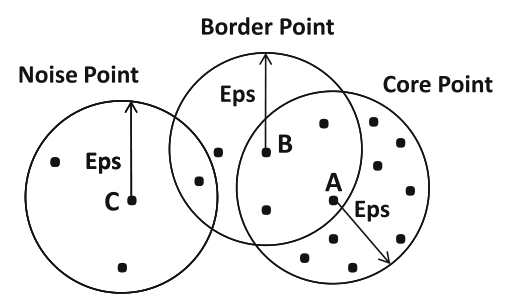Click

## DBSCAN¶

{C}
1.function dbscan(X, eps, min_pts):
2.  initialize NV = X # not visited objects
3.  for x in NV:
4.      remove(NV, x) # mark as visited
5.      nbr = neighbours(x, eps) # set of neighbours
6.      if nbr.size < min_pts:
7.          mark_as_noise(x)
8.      else:
9.          C = new_cluster()
10.         expand_cluster(x, nbr, C, eps, min_pts, NV)
11.         yield C

## expand_cluster¶

{C}
1. function expand_cluster(x, nbr, C, eps, min_pts, NV):
3.  for x1 in nbr:
4.      if x1 in NV: # object not visited
5.          remove(NV, x1) # mark as visited
6.          nbr1 = neighbours(x1, eps)
7.          if nbr1.size >= min_pts:
8.              # join sets of neighbours
9.              merge(nbr, nbr_1)
10.     if x1 not in any cluster:
11.         add(x1, C)
In :
interact(dbscan_demo, eps=FloatSlider(min=0.1, max=10, step=0.05, value=1), min_pts=IntSlider(min=2, max=15, step=1, value=5))

Out:
<function __main__.dbscan_demo(eps=1, min_pts=5)>

## Recap¶

• Do not indicate number of clusters
• Arbitrary shapes of clusters
• Identifies outliers
• Problems with varying density

## Failure for varying density¶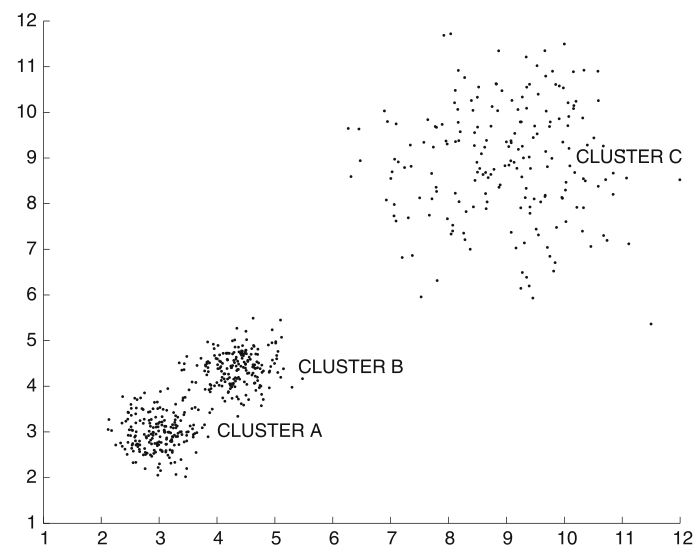• Large min_pts: cluster C is missed
• Small min_pts: clusters A and B get merged

# Cluster Validity and Quality Measures¶

## General approaches¶

• Evaluate using "ground-truth" clustering (Quality Measure)
• invariant to cluster naming
• Unsupervised criterion (Cluster Validity)
• based on intuition:
• objects from same cluster should be similar
• objects from different clusters should be different

## Based on ground-truth¶

#### Rand Index¶

$$\text{Rand}(\hat{\pi},\pi^*) = \frac{a + d}{a + b + c + d} \text{,}$$

where

• $a$ - number of pairs that are grouped both in $\hat{\pi}$ and
• $d$ - number of pairs that are separated both in $\hat{\pi}$ and $\pi^*$ $\pi^*$,
• $b$ ($c$) - number of pairs that are separated both in $\hat{\pi}$ ($\pi^*$), but grouped in $\pi^*$ ($\hat{\pi}$)

#### Rand Index¶

$$\text{Rand}(\hat{\pi},\pi^*) = \frac{tp + tn}{tp + fp + fn + tn} \text{,}$$

where

• $tp$ - number of pairs that are grouped both in $\hat{\pi}$ and
• $tn$ - number of pairs that are separated both in $\hat{\pi}$ and $\pi^*$ $\pi^*$,
• $fp$ ($fn$) - number of pairs that are separated both in $\hat{\pi}$ ($\pi^*$), but grouped in $\pi^*$ ($\hat{\pi}$)

$$\text{ARI}(\hat{\pi},\pi^*) = \frac{\text{Rand}(\hat{\pi},\pi^*) - \text{Expected}}{\text{Max} - \text{Expected}}$$

## Precision, Recall, F-measure¶

• $\text{Precision}(\hat{\pi},\pi^*) = \frac{tp}{tp+fn}$
• $\text{Recall}(\hat{\pi},\pi^*) = \frac{tp}{tp+fp}$
• $\text{F-measure}(\hat{\pi},\pi^*) = \frac{2\cdot Precision \cdot Recall}{Precision + Recall}$

## Cluster validity¶

• Intuition
• objects from same cluster should be similar
• objects from different clusters should be different

### Silhouette¶

For each object $x_{i}$ define:

• $s_{i}$-mean distance to objects in the same cluster
• $d_{i}$-mean distance to objects in the next nearest cluster Silhouette coefficient for $x_{i}$: $$Silhouette_{i}=\frac{d_{i}-s_{i}}{\max\{d_{i},s_{i}\}}$$

Silhouette coefficient for $x_{1},...x_{N}$: $$Silhouette=\frac{1}{N}\sum_{i=1}^{N}\frac{d_{i}-s_{i}}{\max\{d_{i},s_{i}\}}$$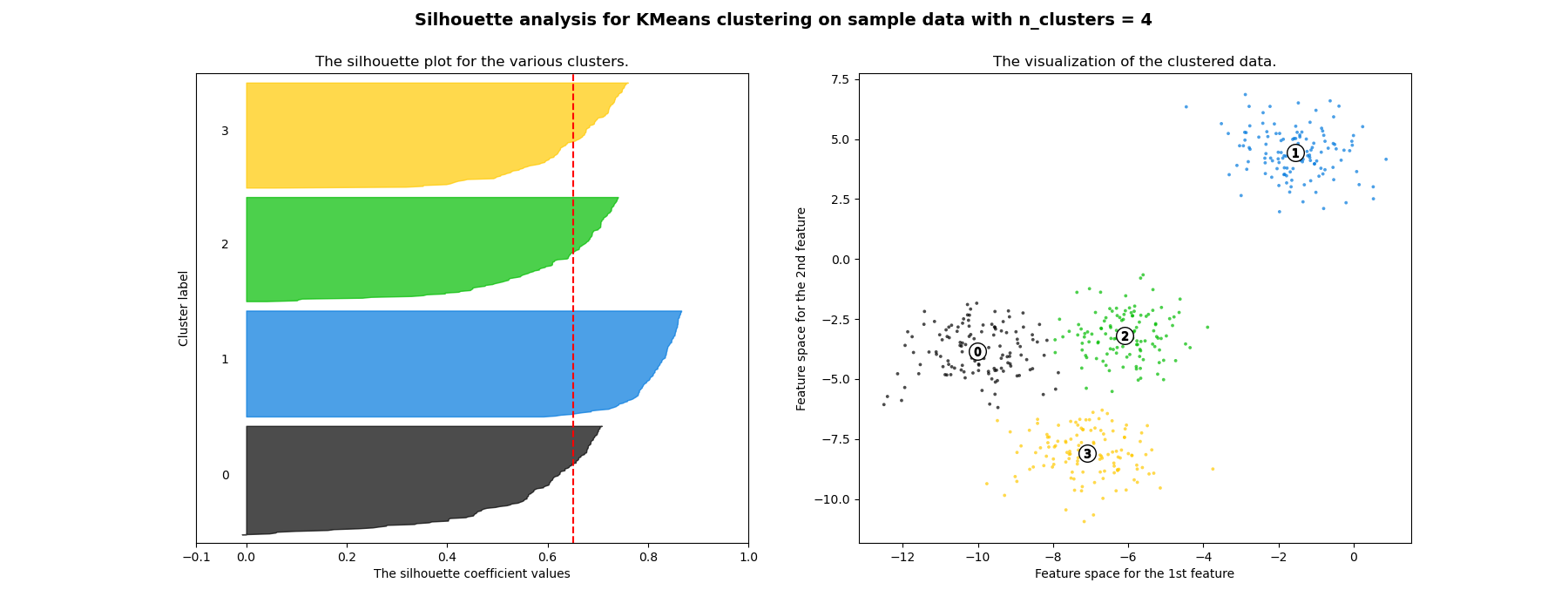## Discussion¶

• The score is bounded between -1 for incorrect clustering and +1 for highly dense clustering.
• Scores around zero indicate overlapping clusters.
• The score is higher when clusters are dense and well separated.
• complexity $O(N^{2}D)$